###DIVISION EXAMPLES

The Bead Unbaffled - An Abacus Manual

Please note that normally the abacus committee suggests leaving 4 empty rod in between dividend and divisor. In some of these examples the constraints of using a small cyber soroban (please overlook the alliteration) dictate that I not follow this rule as closely as I normally would.

Example: 144 ÷ 3  = 48

In this example, the dividend has three whole numbers. Choose E as the unit rod and count three rods to the left. The divisor has one whole number so count one plus two back to the right. This brings us back to rod E. Place the first number of the dividend on rod E.

Step 1: Set the dividend 144 on rods EFG and the divisor 3 on B. (Fig.1)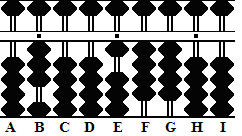Fig.1 ```Step 1 A B C D E F G H I . . .   0 3 0 0 1 4 4 0 0 ```

Step 2:
The divisor (3 on B) is larger than the dividend (1 on E). Therefore apply  Rule II  and set the first number in the quotient one rod to the left of the dividend, in this case on rod D. Divide 3 on B into 14 on rods EF and set the quotient 4 on rod D.

2a: Multiply the quotient 4 on D by 3 on B and subtract the product 12 from rods EF. This leaves the partial quotient 4 on D and the remainder of the dividend 24 on rods FG. (Fig.2)Fig.2 ```Step 2 A B C D E F G H I . . .   0 3 0 0 1 4 4 0 0 (4) Step 2 - 1 2 Step 2a 0 3 0 4 0 2 4 0 0 ```

Step 3: Divide 3 on B into 24 on rods EF and set the quotient 8 on rod E.

3a and the answer: Multiply the quotient 8 on E by 3 on B and subtract the product 24 from rods EF. This leaves the answer 48 on rods DE.  (Fig.3)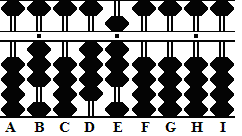Fig.3 ```Step 3 A B C D E F G H I . . .   0 3 0 4 0 2 4 0 0 (8) Step 3 - 2 4 Step 3a 0 3 0 4 8 0 0 0 0 ```

Example: 3,869 ÷ 53  = 73

In this example, the dividend has four whole numbers. Choose rod E as the unit and count four rods to the left. The divisor has two whole numbers so count two plus two back to the right. This brings us to back rod E. Place the first number of the dividend on rod E.

Step 1: Set the dividend 3869 on rods EFGH and the divisor 53 on AB. (Fig.4)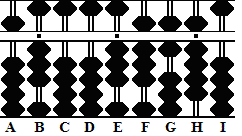Fig.4 ```Step 1 A B C D E F G H I . . .   5 3 0 0 3 8 6 9 0 ```

Step 2:
Because the first two digits in the divisor are larger than those of the dividend apply  Rule II  and begin by placing the quotient one rod to the left of the dividend, in this case on rod D. Divide 5 on A into the 38 on rods EF and set the quotient 7 on rod D.

2a: Multiply 7 on D by the 5 on A and subtract the product 35 from 38 on rods EF.

2b: Multiply 7 on D by the 3 on B and subtract the product 21 from 36 on rods FG. This leaves the partial quotient 7 on rod D and the remainder of the dividend 159 on rods FGH. (Fig.5)Fig.5 ```Step 2 A B C D E F G H I . . .   5 3 0 0 3 8 6 9 0 (7) Step 2 - 3 5 Step 2a 5 3 0 7 0 3 6 9 0 - 2 1 Step 2b 5 3 0 7 0 1 5 9 0 ```

Step 3: Divide 5 on A into 15 on FG. Set the quotient 3 on Rod E.

3a: Multiply 3 on E by the 5 on A and subtract the product 15 from 15 on rods EF.

3b and the answer: Multiply 3 on E by 3 on B and subtract the product 9 from rod H. This leaves the answer 73 on rods DE. (Fig.6)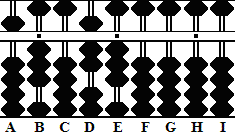Fig.6 ```Step 3 A B C D E F G H I . . .   5 3 0 7 0 1 5 9 0 (3) Step 3 - 1 5 Step 3a 5 3 0 7 3 0 0 9 0 - 9 Step 3b 5 3 0 7 3 0 0 0 0 ```

Example: 0.54 ÷ 3.6  = 0.15

In this example, choose rod E as the unit rod. The dividend has no whole numbers, so there's no counting to the left. The divisor has one whole number so count one plus two to the right. This brings us to rod H. Place the first number of the dividend on rod H.

Step 1: Set the dividend 54 on rods HI and the divisor 36 on BC. (Fig.7)Fig.7 ```Step 1 A B C D E F G H I . . .   0 3 6 0 0 0 0 5 4 ```

Step 2:
Because the first two digits in the divisor are smaller than those of the dividend apply  Rule I and begin by placing the quotient two rods to the left of the dividend, in this case on rod F. Divide 3 on B into the 5 on rod H and set the quotient 1 on rod F.

2a: Multiply 1 on F by the 3 on B and subtract the product 3 from 5 on rod H.

2b: Multiply 1 on F by the 6 on C and subtract the product 6 from 14 on rods HI. This leaves the partial quotient 1 on rod F and the remainder of the dividend 18 on rods HI. (Fig.8)Fig.8 ```Step 2 A B C D E F G H I . . .   0 3 6 0 0 0 0 5 4 (1) Step 2 - 3 Step 2a 0 3 6 0 0 1 0 2 4 - 6 Step 2b 0 3 6 0 0 1 0 1 8 ```

Step 3: Divide 3 on B into 18 on HI. It looks like the answer should be 6. However, in order to continue working the problem there must be a remainder. Instead use the quotient 5. Set 5 on rod G.

3a: Multiply 5 on G by the 3 on B and subtract the product 15 from 18 on rods HI.

3b and the answer: Multiply 5 on G by 6 on C and subtract the product 3 (of 30) from rod I. This leaves 15 on rods FG. Because we designated rod E as the unit rod the answer appears on the soroban as 0.15 (Fig.9)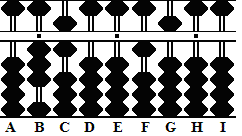Fig.9 ```Step 3 A B C D E F G H I . . .   0 3 6 0 0 1 0 1 8 (5) Step 3 - 1 5 Step 3a 0 3 6 0 0 1 5 0 3 - 3 Step 3b 0 3 6 0 0 1 5 0 0 ```

Example: 20956 ÷ 403  = 52

This is a good example because there are zeros in both the divisor and dividend. Division problems of this type can be confusing to those new to soroban work.

In this example the dividend has five whole numbers. Choose rod H as the unit and count five rods to the left. The divisor has three whole numbers so count three plus two back to the right. This brings us to back rod H. Place the first number of the dividend on rod H.

Step 1: Set the dividend 20956 on rods HIJKL and the divisor 403 on rods CDE (Fig.10)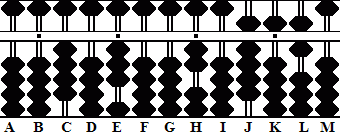Fig.10 ```Step 1 A B C D E F G H I J K L M . . . .    0 0 4 0 3 0 0 2 0 9 5 6 0 ```

Step 2:
The divisor (4 on C) is larger than the dividend (2 on H). Therefore apply Rule II  and set the first number in the quotient one rod to the left of the dividend on rod G. Divide 4 on C into 20 on rods HI and set the quotient 5 on rod G.

2a: Multiply 5 on G by 4 on C and subtract the product 20 from rods HI.

2b: Multiply the quotient 5 on G by 3 on E.  Remembering to take the zero in the divisor into account, skip one rod to the right and subtract the product 15 from rods JK. This leaves the partial quotient 5 on G and the remainder of the dividend 806 on rods JKL. (Fig.11)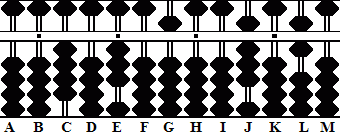Fig.11 ```Step 2 A B C D E F G H I J K L M . . . .    0 0 4 0 3 0 0 2 0 9 5 6 0 (5) Step 2 - 2 0 Step 2a 0 0 4 0 3 0 5 0 0 9 5 6 0 - 1 5 Step 2b 0 0 4 0 3 0 5 0 0 8 0 6 0 ```

Step 3: Divide 4 on C into 8 on rod J and set the quotient 2 on rod H.

3b:  Multiply 2 on H by 4 on C and subtract 8 from rod J.

3b and the answer:  Multiply the quotient 2 on H by 3 on E. Once again, because of the zero in the divisor skip one rod to the right and subtract the product 6 from rod L leaving the answer 52 on rods GH. (Fig.12)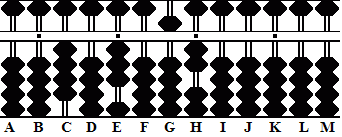Fig.12 ```Step 3 A B C D E F G H I J K L M . . . .    0 0 4 0 3 0 5 0 0 8 0 6 0 (2) - 8 Step 3 0 0 4 0 3 0 5 2 0 0 0 6 0 - 6 Step 3a 0 0 4 0 3 0 5 2 0 0 0 0 0 ```

 Here's a link to another example: 3.908 ÷ 0.39 = 10.0205128

Print Page (.pdf format,118kb)